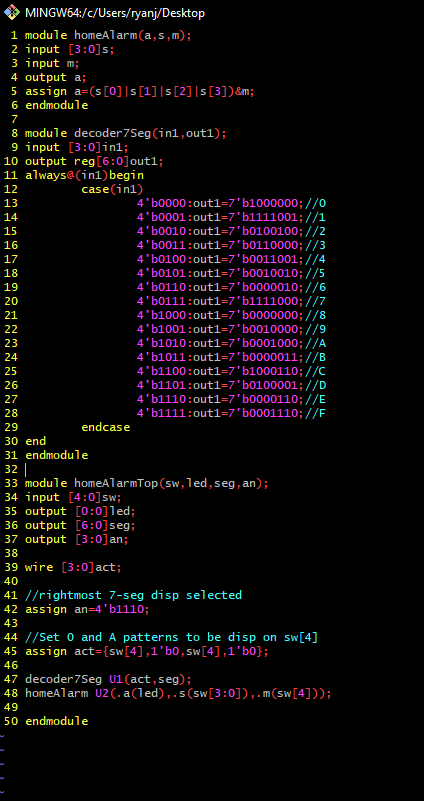CE433 Embedded Devices Spring 2022
Homework 3 - Combinational Logic Blocks
Name: Ryan Jeanes

Email: rejeanes@fortlewis.edu

Homework 3 - Combinational Logic Blocks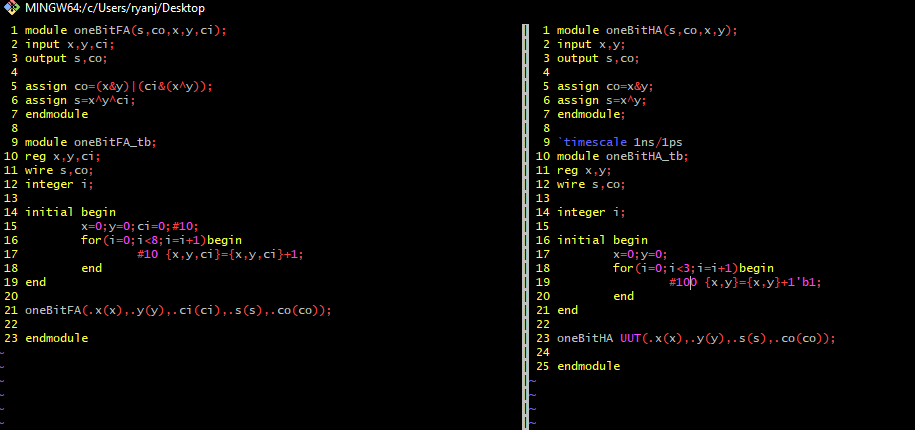Figure 1 - Verilog code for both the one bit FA and one bit HA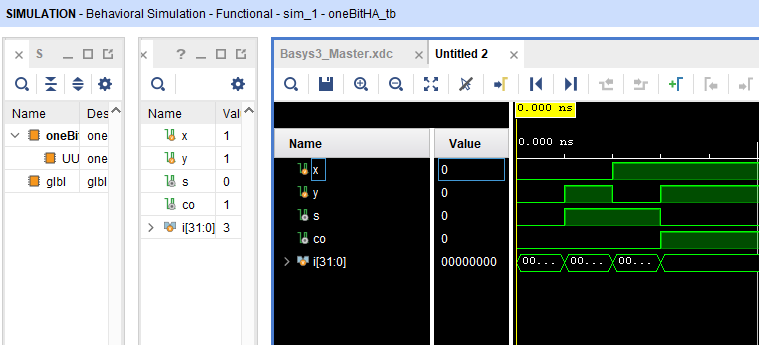Figure 2 - Sim of the one bit HA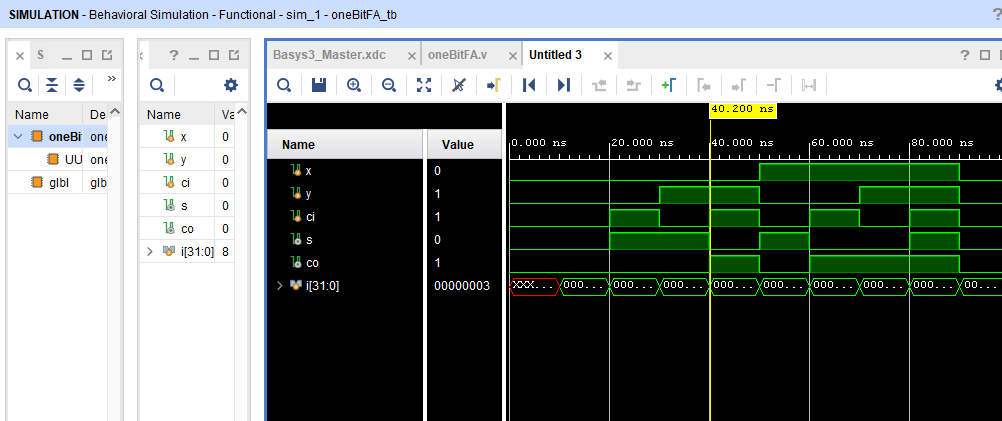Figure 3 - Sim of the one bit FAFigure 4 - Code for 1 bit comparator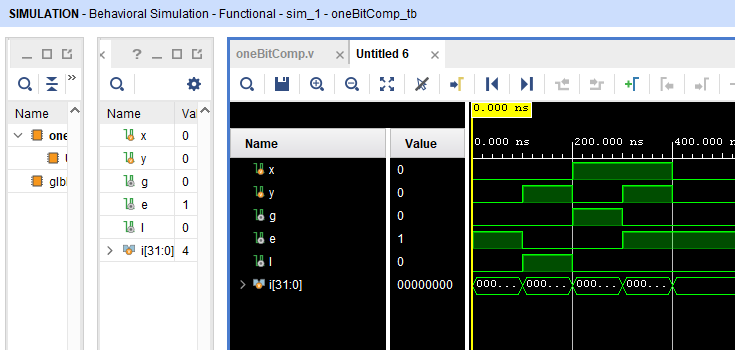Figure 5 - 1 bit comparator simulationFigure 6 - Code for 4 bit comparator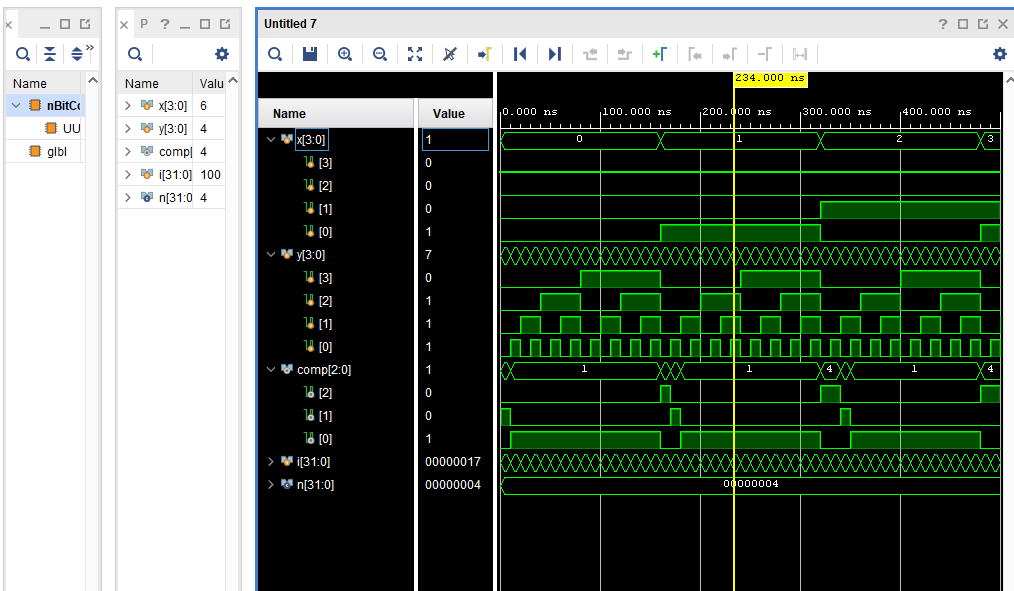Figure 7 - Simulation for 4 bit comparatorFigure 8 - Code for 2 bit comparator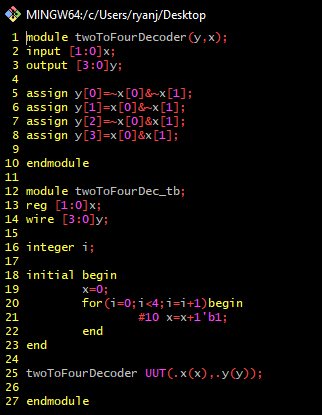Figure 9 - Code for 2-4 decoder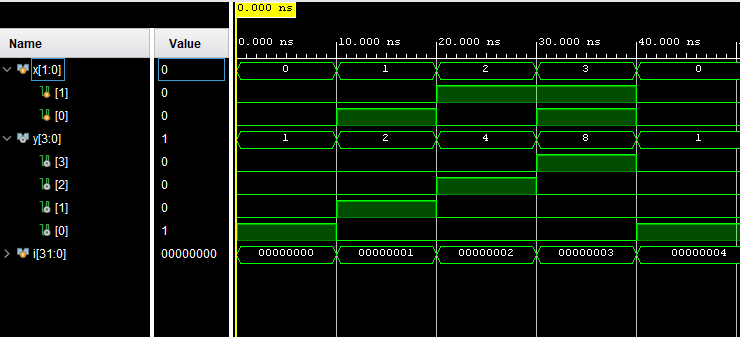Figure 10 - Simulation for the 2-4 decoder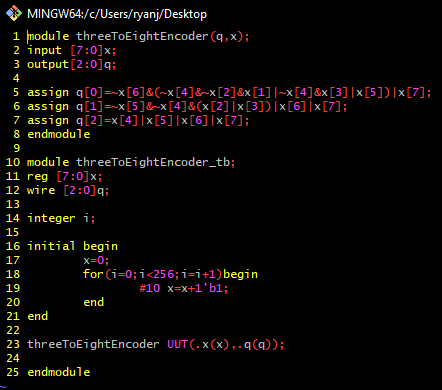Figure 11 - Code for 8x3 priority encoder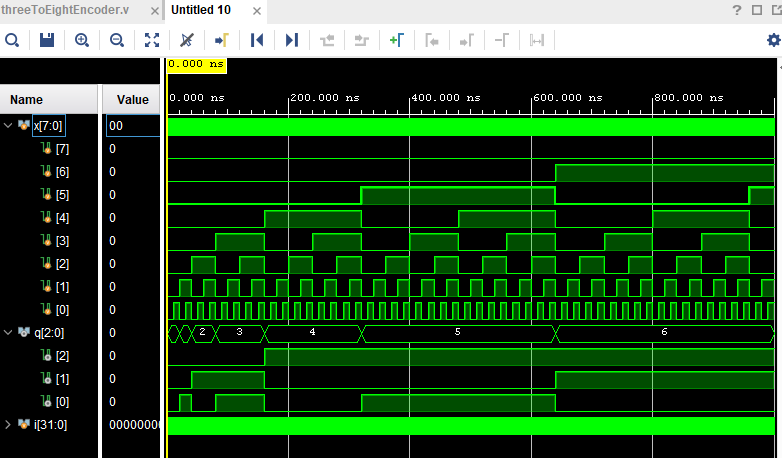Figure 12 - Simulation for 8x3 priority encoder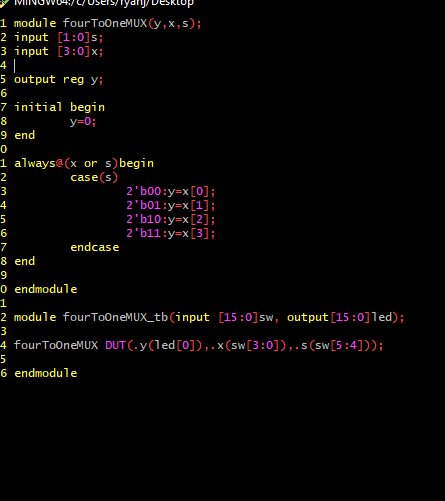Figure 12 - Simulation for 8x3 priority encoder

<iframe width="420" height="315" src="https://youtu.be/b1OxyzzpQx4" frameborder="0" allowfullscreen></iframe>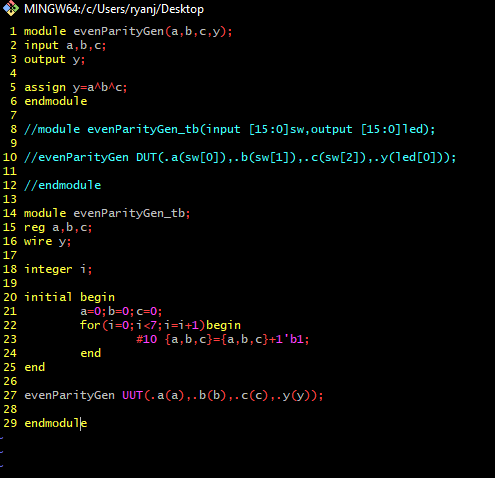Figure 13 - Even parity generator
code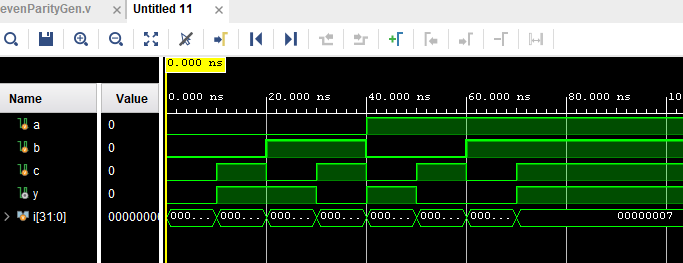Figure 14 - Simulator for even parity generator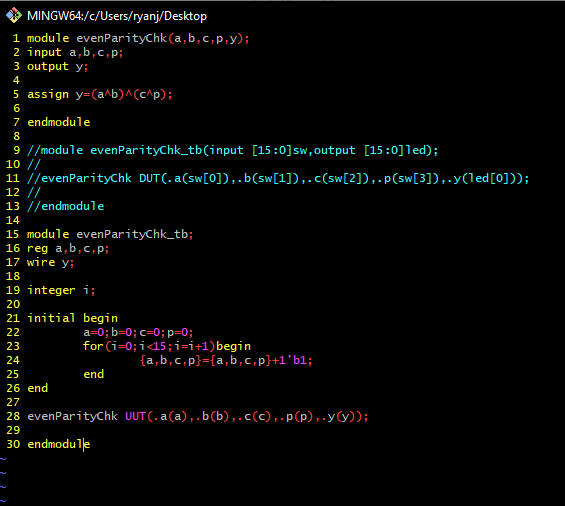Figure 15 - Code for even parity checker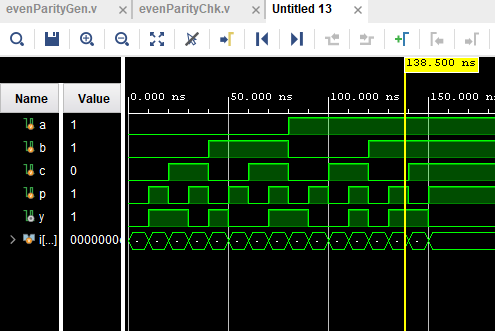Figure 16 - Simulation of even parity checker

I cannot get embeds to work, Kompozer keeps grinding to a halt along with spamming me with seemingly endless server certification warnings every time I try to implement the HTML code for video embedding.

So here is the even parity generator
Here is the even parity checker